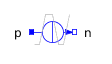Proximity - MapleSim Help

Proximity

Proximity sensor between two frames.Description The equations for the Proximity component are given below. Distance: $\mathbf{r}={\mathbf{r}}_{b}\mathbf{-}{\mathbf{r}}_{a}=⟨\left({x}_{b}-{x}_{a}\right),\left({y}_{b}-{y}_{a}\right),\left({z}_{b}-{z}_{a}\right)⟩$, is the relative displacement vector.   The distance ($\mathrm{dist}$) is calculated based on the  and $\mathrm{e__axis}$  parameters:   Trigger: The output signal, $\mathrm{proximity}$, becomes true when:Connections

 Name Description Modelica ID ${\mathrm{frame}}_{a}$ Inboard frame frame_a ${\mathrm{frame}}_{b}$ Outboard frame frame_b $\mathrm{proximity}$ Boolean output signal proximity $\mathrm{reset}$ Optional boolean input signal resetParameters

 Symbol Default Units Description Modelica IDWhen checked (true) the distance is calculated along the selected axis of frame_a. useAxis $\mathrm{δ}$ 1e-3 [m] Distance threshold between frame_a and frame_b to trigger the sensor. delta $\mathrm{e__axis}$ [1,0,0] Unit vector (in frame_a) for distance calculation. e_axis $\mathrm{Hold}$When checked (true) the output will remain true after the first trigger. When unchecked (false) the output turns to false as soon as the distance between the frames becomes greater than the given threshold ($\mathrm{δ}$). holdWhen checked (true) an input is enabled to reset the sensor. useReset